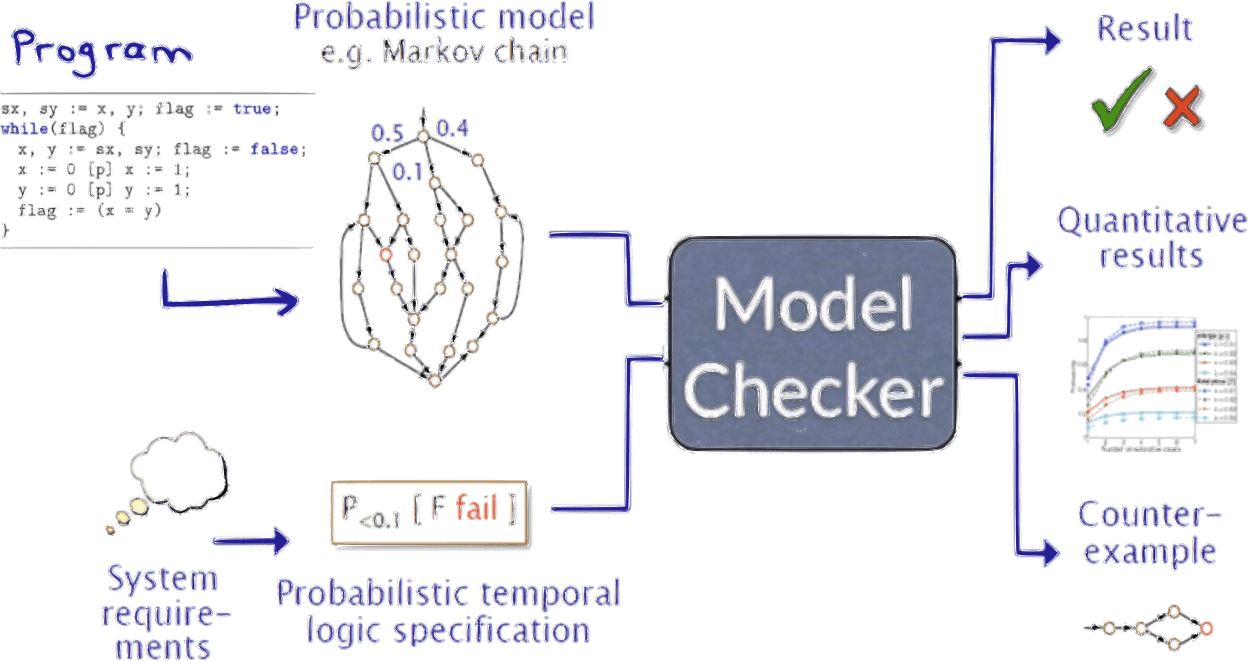Probabilistic Model Checking with Storm

A 1-day workshop introducing the concept of probabilistic model checking and its applications with the Storm probabilistic model checker.

$$\mathop{Pr}_{🌍}(1 U \checkmark \to \neg(1 U 😔)) = 1 \hspace{1cm} \text{(unproven claim)}$$

Many systems in the real world are inherently probabilistic. Verifying correctness, safety, and reliability of such systems is a challenging task that involves reasoning about their behavior in time.

• What is my best option to get to the airport?
• Will an electric car be able to reach its destination within the remaining battery capacity?
• How long will it take for a robot to complete a task?

All these questions involve probabilistic reasoning about the behavior of a system.

Markov chains (MCs) and Markov decision processes (MDPs) are popular formalisms for modeling such systems. Often, answering the above questions reduces to computing the (extremal) probability of a possibly complex event. Temporal logics such as linear time logic (LTL) or computational tree logic (CTL) are capable of describing such events and can be used to formulate queries about the behavior of a system. Together, MCs, MDPs, and temporal logics form the basis of probabilistic model checking (PMC). With its formal setup, PMC aims for efficient computation of sound quantitative results. As a useful side product many approaches allow generating counterexamples in case that a specification is violated. PMC therefore provides a powerful tool for verifying the correctness of systems.MDP model of an airport journey

In this workshop, we will introduce the formal concept of probabilistic model checking. We review the potential and the limitations and demonstrate practical application with the Storm probabilistic model checker. The content is based on the UAI 2022 tutorial by professor Dr. Joost-Pieter Katoen and Dr. Sebastian Junges, two of the main researchers behind the library.

# Learning outcomes

• Understand the theory behind probabilistic model checking.
• Get to know the most important algorithms
• Learn how to model systems as Markov chains and Markov decision processes.
• Learn how to formulate queries about the behavior of a system.
• Learn how to use the Storm probabilistic model checker.

# Structure of the workshopA multi objective query in LTL

## Part 1: Fundamentals of Probabilistic Model Checking

We will start with an introduction into the basics of probabilistic model checking. We will discuss the important notions of Markov chains and Markov decision processes and define the semantics of the temporal logics LTL and CTL.

• Markov chains and Markov decision processes.
• LTL and CTL.

## Part 2: Hands-on probabilistic Model Checking with StormEfficient probabilistic model checking We will then move on to a hands-on session where we will use the Storm probabilistic model checker to verify properties of some simple models.

• Storm probabilistic model checker

## Part 3: Parameter Synthesis in Markov Models.Sound quantitative results So far, we have only considered the case where the parameters of a Markov model are known. In many applications, however, some parameters are free to choose. In this part, we will discuss how to synthesize parameters of a Markov model such that a given specification is satisfied with high probability.

• Parameter synthesis in Markov models.

# Prerequisites

• Basic knowledge of probability theory.
• no particular programming skills are required.
• No prior knowledge of machine learning is required.
• The probabilistic model checker Storm, Christian Hensel, Sebastian Junges, Joost-Pieter Katoen, Tim Quatmann, Matthias Volk. International Journal on Software Tools for Technology Transfer (2022)
• The Probabilistic Model Checking Landscape, Joost-Pieter Katoen. Proceedings of the 31st Annual ACM/IEEE Symposium on Logic in Computer Science (2016)